# 9.3. Dynamic Memory Allocation of 2D Arrays#

We discussed in Chapter 8 the dynamic memory allocation of 1D arrays. We said it is necessary to use when:

1. We do not know the number of elements in an array before run-time, for example, the array size is taken as user input, or based on a calculation happening at run-time. It is better to allocate the array dynamically as this will allow us to check if the array was allocated or not on the heap. If heap does not have enough space, malloc returns NULL.

2. We want the life-time of the array to be flexible and not dependent on the scope of the array. For example, if you declare an array in a function using int arr;, the memory space for it will be destroyed when we return from the function. This is because arr variable was created on the stack. However, if you need the space of the array elsewhere in another function, you can always pass a pointer to the dynamically allocated space. The space of the array will only be destroyed by free function.

To review dynamic memory allocation for 1D array, please see Chapter 8.1.

The question that we tackle in this section is how can we dynamically allocate a 2D array. There are three ways to do so. We will discuss one method in detail, and the other two are quite intuitive.

For example, let’s dynamically allocate a 2D array of integers with 3 rows and 4 columns that looks like Fig. 9.6.

## 9.3.1. Method 1: Dynamic Allocation of an array of pointers#

The first method to dynamically allocate a 2D array is to allocate an array of pointers, and then have each of these pointers point to a dynamically allocated 1D array corresponding to a row in the 2D array.

Step 1: Dynamically Allocate an Array of Rows Pointers. First, we dynamically allocate an array of pointers, where each of these pointers will later point to a row in the 2D array. Hence, the number of pointers we need is equal to the number of rows, which is $$3$$ in this example. Fig. 9.7 shows what we need to allocate and the pointer to the first element in the array will be arr.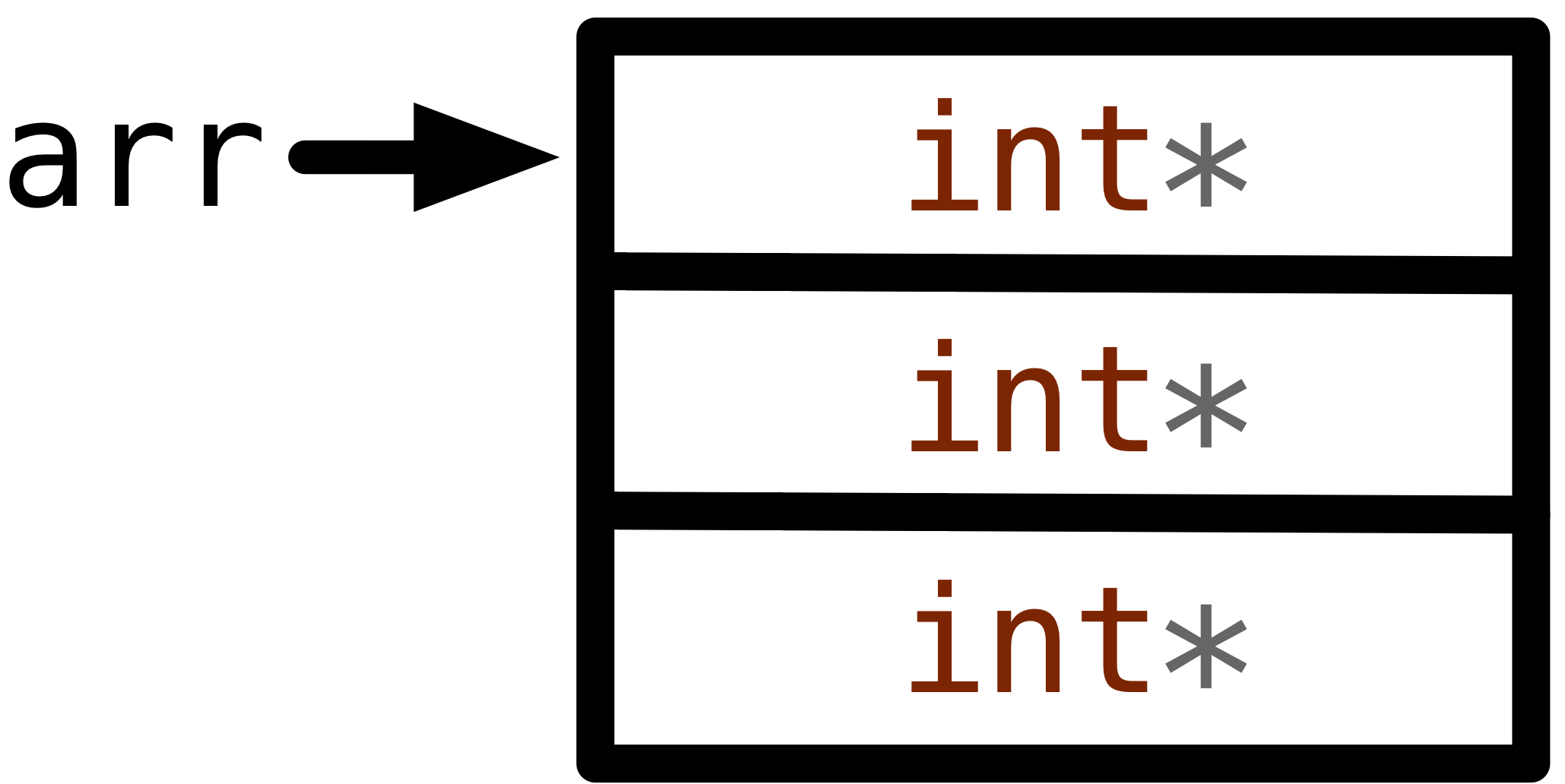Fig. 9.7 Dynamically allocating a 1D array of pointers pointed to by arr.#

To do what Fig. 9.7 requires, we need the following statement: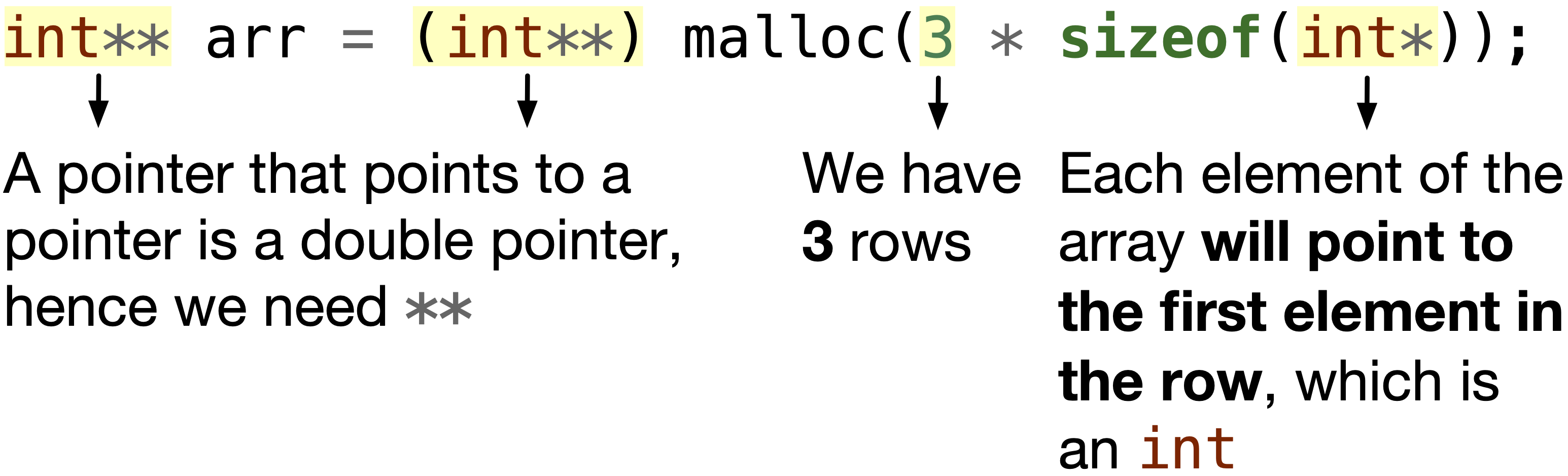Fig. 9.8 The statement to dynamically allocate a 1D array of pointers pointed to by arr.#

Step 2: Dynamically Allocate Each Row. Second, we dynamically allocate 1D arrays each corresponding to a row. We need to have each element in Fig. 9.7 to point to each row as shown in Fig. 9.9.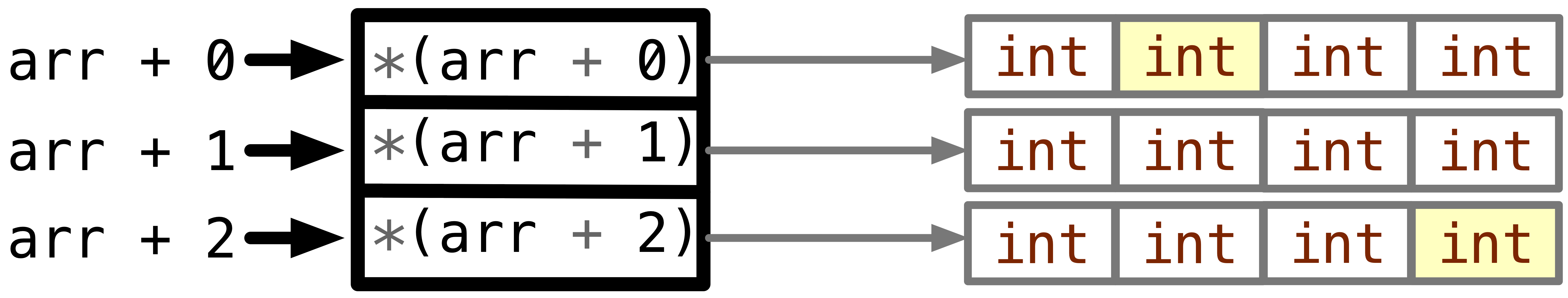Fig. 9.9 Each int* in the array should point to a 1D array having elements of a row.#

We can do so using the following for-loop in Fig. 9.10.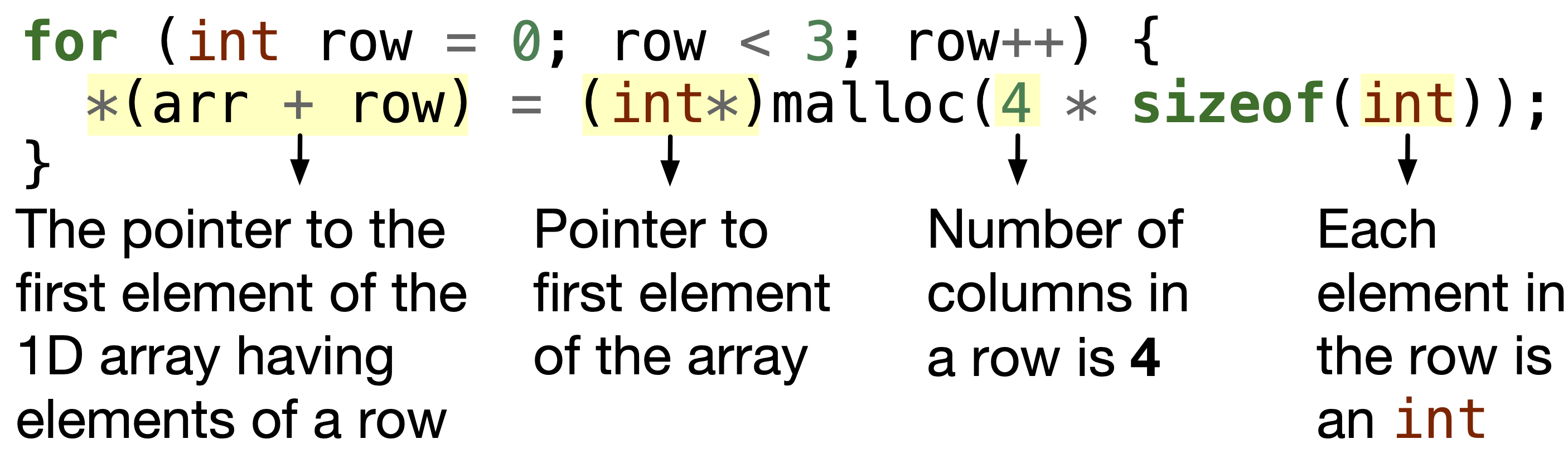Fig. 9.10 For each row, dynamically allocate 1D array having number of columns elements.#

Note: It may be obvious to you that instead of *(arr + row), you can also write it as arr[row].

Step 3: Assign a Value to Each Element.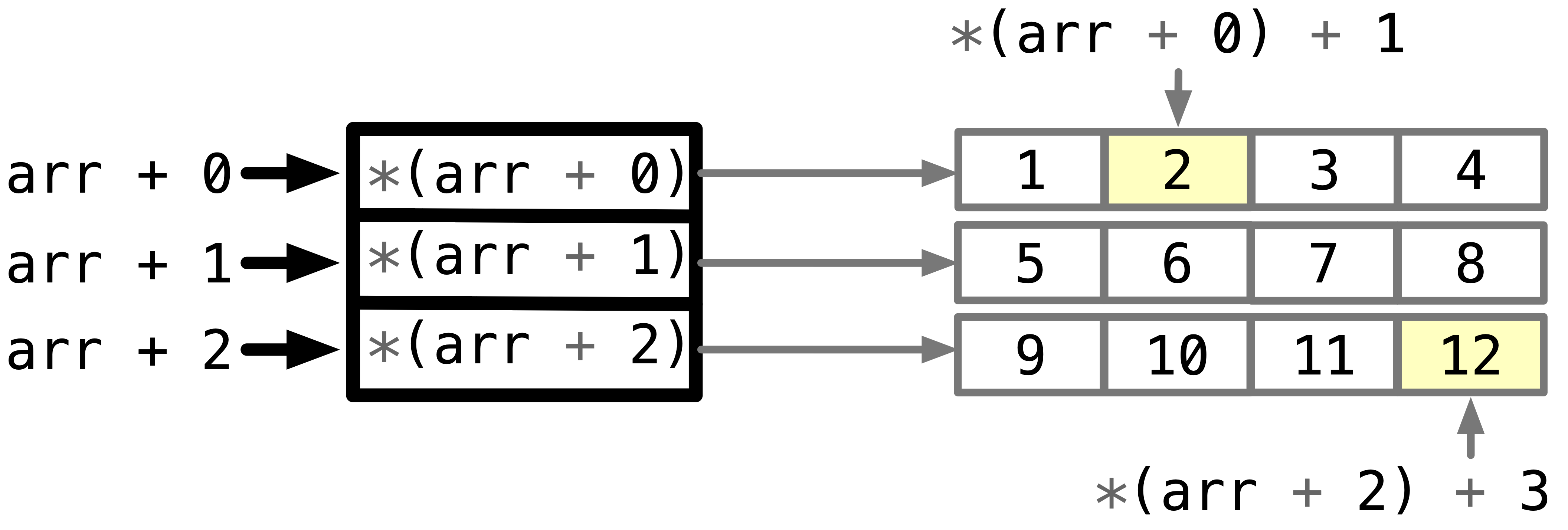Fig. 9.11 To access the element at row index $$0$$ and column index $$1$$, you can either access it using *(*(arr + 0) + 1) or arr.#

We can access each element in a nested for loop as shown in Fig. 9.12.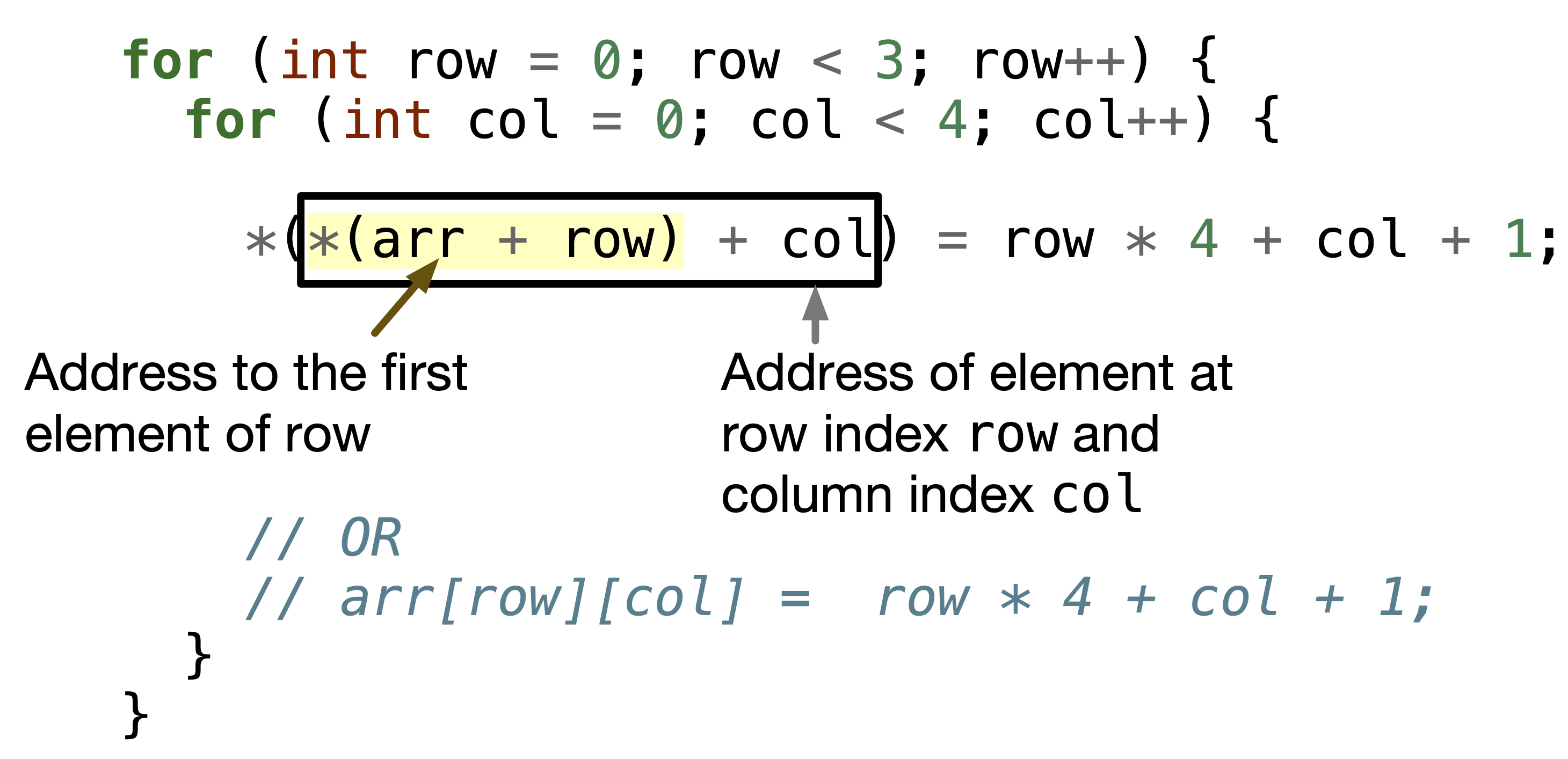Fig. 9.12 To access each element in the 2D array, you can either access it using *(*(arr + row) + col) or arr[row][col].#

Step 4: Free Dynamically Allocated Space. To deallocate or free the dynamically allocated space, we need to free the space. We need to take care of the order of deallocation and number of deallocations.

If we were to only do free(arr) only, we will still have the dynamically allocated three 1D arrays.

Another incorrect way is to free(arr) first, then free(*(arr + 0)), free(*(arr + 1)) and free(*(arr + 2)). It is incorrect because we cannot access *(arr + 1) after free(arr), since arr is freed.

The correct way is to free the three 1D arrays using free(*(arr + 0)), free(*(arr + 1)) and free(*(arr + 2)), then free(arr) as shown in the following figure.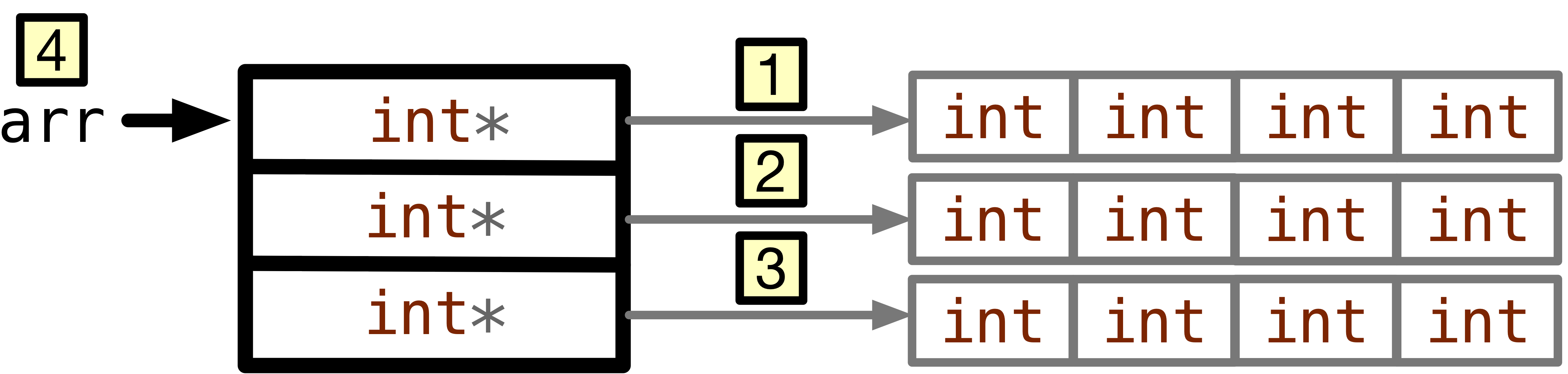Fig. 9.13 The correct way is to free the three 1D arrays then the array of pointers.#

Code Snippet

for (int row = 0; row < 3; row++) {
free(*(arr + row));
// OR
// free(arr[row]);
arr[row] = NULL;
}

free(arr);
arr = NULL;


Download 2D-dyn-mem-alloc.c if you want to run the following program yourself.

Code


#include <stdlib.h>

int main(void) {
// Dynamically allocate array of pointer
int** arr = (int**)malloc(3 * sizeof(int*));

// Dynamically allocate a 1D array for each row
for (int row = 0; row < 3; row++) {
*(arr + row) = (int*)malloc(4 * sizeof(int));
}

// Assign a value to each element
for (int row = 0; row < 3; row++) {
for (int col = 0; col < 4; col++) {
*(*(arr + row) + col) = row * 4 + col + 1;
// arr[row][col] =  row * 4 + col + 1;
}
}

// Free the 1D arrays of rows first
for (int row = 0; row < 3; row++) {
free(*(arr + row));
// OR
// free(arr[row]);
arr[row] = NULL;
}

// Then free the array of pointers
free(arr);
arr = NULL;

return 0;
}



## 9.3.2. Method 2: Static Allocation of an array of pointers#

First, statically allocate an array of pointers int* arr;. The problem is this array will be on the stack, hence number of rows has to be known at compile-time. While in method 1, all the elements were on the heap except for arr pointer.

Second, make each of these pointers in the array point to dynamically allocated 1D array corresponding to the row.

Third, we can access the elements as any 2D array.

Forth, we free only the dynamically allocated three 1D arrays.


#include <stdlib.h>

int main(void) {
// on Stack, statically have allocate 3 pointers,
// each will point to a 1D array corresponding to a row
int* arr;

// Access each pointer and make it point
// to a newly dynamically allocated array
for (int row = 0; row < 3; row++) {
arr[row] = (int*)malloc(4 * sizeof(int));
}
// Access elements as usual
for (int row = 0; row < 3; row++) {
for (int col = 0; col < 4; col++) {
arr[row][col] = row * 4 + col + 1;
}
}
// Free only dynamically allocated space
for (int row = 0; row < 3; row++) {
free(arr[row]);
}

return 0;
}



## 9.3.3. Method 3: Dynamic Allocation of a 1D array#

The last method is easy if you understand from Chapter 9: Section 9.1.2 how does a static 2D array looks like in the memory.

We can dynamically allocate 1D array having rows $$\times$$ columns integer elements = $$12$$.

To access elements, you cannot use arr[row][col], because the number of the columns is unknown. Instead, you need to do *(arr + row * cols + col) to access the element at index row and column index col when the number of columns is cols.

Finally, to free the dynamically allocated space, you only need to free(arr); which is a 1D array of int.


#include <stdlib.h>

int main(void) {
int rows = 3, cols = 4;
int* arr = (int*)malloc(rows * cols * sizeof(int));

for (int row = 0; row < rows; row++) {
for (int col = 0; col < cols; col++) {
*(arr + row * cols + col) = row * cols + col + 1;
}
}

free(arr);

return 0;
}



0 Questions

X